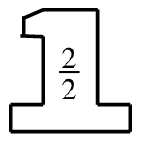### Home > CC2 > Chapter 1 > Lesson 1.2.6 > Problem1-115

1-115.

Fareed wants to add $\frac { 1 } { 4 } + \frac { 5 } { 8 }$.

1. Add the fractions by using a Giant One to create a common denominator.

What do you need to multiply the first term by so that the denominator is equal to $8$?

Multiplying the denominator by $2$ gives us $8$. Now use a Giant One to solve the problem.

$\frac{1}{4} \:\cdot$$=\frac{2}{8}$

Now that both fractions have the same denominator, you can add the fractions together by adding the numerator and keeping the denominator.

$\frac{2}{8}+\frac{5}{8}=?$

$\frac{7}{8}$